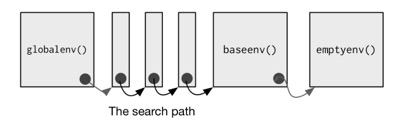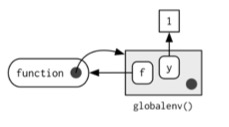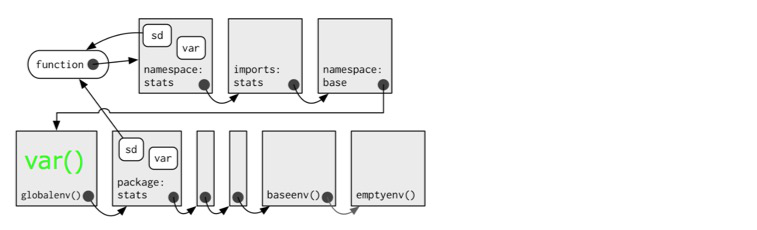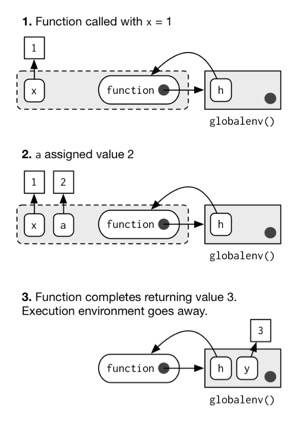# Advanced R: Environments tutorial

## Basics

• Every environment except the empty environment, the ultimate ancestor of all environments, has a parent.
• If a name is not found in an environment, then R will look in its parent (and so on).`globalenv()`
the environment in which you normally work.
`baseenv()`
the environment of the `base` package. Its parent is the `empty` environment.
`emptyenv()`
is the ultimate ancestor of all environments
Simple operations related to environments
``````e <- new.env()
parent.env(e)
e\$a <- 1
e\$b <- 2
e\$c <- 3
ls(e)
str(e)
ls.str(e)
get("c", envir = e)
rm("a", envir = e)
ls(e)
exists("a", envir = e)
exists("b", envir = e)
identical(globalenv(), environment())``````
``````<environment: R_GlobalEnv>
 "a" "b" "c"
<environment: 0x7fd053291808>
a :  num 1
b :  num 2
c :  num 3
 3
 "b" "c"
 FALSE
 TRUE
 TRUE
``````

## Function environments

There are different kinds of environments related to functions

binding environment
binding a function to a name with `<-` defines a binding environment
execution environment
calling a function creates an ephemeral execution environment that stores variables created during execution
calling environment
Every execution environment is associated with a calling environment, which tells you where the function was called

### The enclosing environment

• The environment where the function was created.
``````y <- 1
f <- function(x) x + y``````In this case, globalenv() is both the enclosing and the binding environment.

### Binding environments

``````e <- new.env()
e\$g <- function() 1``````globalenv() is the enclosing, e is the binding

### Package Namespaces

This works because every package has two environments associated with it: the package environment and the namespace environment.

package environment
every publicly accessible function, and is placed on the search path.
namespace environment
contains all functions (including internal functions), and its parent environment is a special imports environment that contains bindings to all the functions that the package needs.

Every exported function in a package is bound into the package environment, but enclosed by the namespace environment. This complicated relationship is illustrated by the following diagram:When we type var (green) into the console, it's found first in the global environment(which is one of binding environments). When sd() looks for var() it finds it first in its namespace environment so never looks in the globalenv().

### Execution environments

• Every time a function is called, a new environment is created to host execution.
• The parent of the execution environment is the enclosing environment of the function.
• Once the function has completed, this environment is thrown away.When you create a function inside another function,

• The enclosing environment of the child function is the execution environment of the parent
• which means the execution environment is no longer ephemeral.

### Calling environments

• R’s regular scoping rules only use the enclosing parent
• `parent.frame()` allows you to access the calling parent.
``````x <- 20
f <- function() {
x <- 10
def <- get("x", environment())
cll <- get("x", parent.frame())
print(def)
print(cll)
}
f()``````
`````` 10
 20
``````

## Biding names to values

• Names usually consist of letters, digits, `.` and `_`, and can’t begin with `_`.
• There are two other special types of binding, delayed and active:
• `<-` creates a variable in the current environment.
• `<<-` modifies an existing variable found by walking up the parent environments. if not found any, creates a variable in `globalenv`.
• A delayed binding creates and stores a promise to evaluate the expression when needed.
• We can create delayed bindings with the special assignment operator `%<d-%`, provided by the `pryr` package.
• Active are not bound to a constant object. Instead, they’re re-computed every time they’re accessed
• To create active binding, use `%<a-\$`.
``````library(pryr)
system.time(b %<d-% {Sys.sleep(1); 1})
system.time(b)
system.time(b)``````
``````   user  system elapsed
0       0       0
user  system elapsed
0       0       1
user  system elapsed
0       0       0
``````
``````library(pryr)
x %<a-% runif(1)
x
x``````
`````` 0.1530638
 0.4654055
``````

## Explicit environments

• Unlike most objects in R, when you modify an environment, it does not make a copy.
• Since environments have reference semantics, you’ll never accidentally create a copy. This makes it a useful vessel for large objects.
``````modify <- function(x) {
x\$a <- 2
invisible()
}``````
the original list is not changed because modifying a list actually creates and modifies a copy.
``````l <- list()
l\$a <- 1
modify(l)
l\$a``````
`````` 1
``````
However, if you apply it to an environment, the original environment is modified:
``````e <- new.env()
e\$a <- 1
modify(e)
e\$a``````
`````` 2
``````

# `search()`reference

`search()` lists all parents of the global environment.

``search()``
`````` ".GlobalEnv"        "package:stats"     "package:graphics"
 "package:grDevices" "package:utils"     "package:datasets"
 "package:methods"   "Autoloads"         "package:base"
``````

# `new.env(parent = parent.frame())`reference

``````e <- new.env()
# The default parent provided by new.env() is environment from
# which it is called - in this case that's the global environment.
# This is because R evaluates function arguments lazily.
parent.env(e)``````
``````<environment: R_GlobalEnv>
``````

# `ls(name, all.names = FALSE)`reference

`name`
this accepts an `environment` object
`all.names`
If `FALSE`, names which begin with a `.` are omitted
``````x = 10
y = 20
.hidden = 30
ls(environment())
ls(environment(), all.names = TRUE)``````
`````` "x" "y"
 ".hidden" "x"       "y"
``````

# `ls.str(name, all.names = FALSE)`reference

Show `str()` of all names from `ls()`

``````x = 10
y = 20
ls.str(environment())``````
``````x :  num 10
y :  num 20
``````

# `parent.env()` vs `parent.frame()`discussion

• `parent.env()` returns the enclosing environment
• `parent.frame()` returns the calling environment
``````name <- "global"
f <- function() {
name <- "f"
g <- function() {
e <- environment()
list(parent.env = get("name", parent.env(e)),
parent.frame = get("name", parent.frame()))
}
}
g <- f()
g()``````
``````\$parent.env
 "f"

\$parent.frame
 "global"
``````

# `within(data, expr, ...)`reference

`data`
data to use for constructing an environment, may be an environment, a list, a data frame, etc.
`expr`
expression to evaluate

Unlike `with()`, `within()` makes a modified copy of `data`. `with()` returns the evaluated `expr`, while `within()` returns the modified copy.

``````e <- new.env()
with(e, x <- 10)
ls.str(e)``````
``````x :  num 10
``````
``````l <- list()
within(l, x <- 20)``````
``````\$x
 20
``````